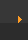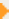## Stoichiometry: Gram Atomic Mass (Page 1 of 4)

### Some Notes about Gram Atomic Mass

• When calculating the gram atomic mass, remember to round to the appropriate number of figures. We round to two figures on this website.
• The number of grams given on the periodic table represents the mass of one mole of that element. For example, when I look at hydrogen on the periodic table the mass is 1.0079. This means that one mole of hydrogen will have a mass of 1.0079 grams. Remember, the GAM tells you the mass of one mole of that specific element.
• In the next section we'll look at molecules (for example H2O) and add up the GAM of all the atoms to find the Gram Formula Mass (GFM) or the mass of one mole of that particular molecule.
 Next pageYou'll need your periodic table (or use www.ptable.com) to complete this section.

GAM stands for Gram Atomic Mass and describes the mass of one mole of an element.

The GAM is found on the periodic table; usually under the symbol for the element.

The units for the GAM are grams/mole. This is abbreviated as g/mol.

On this site we will round to two decimal places (e.g Fe = 55.85 g/mol).